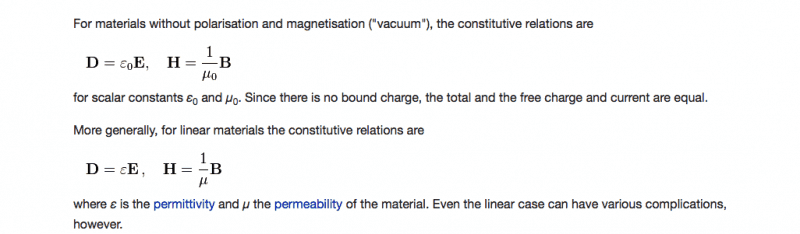# Maxwell's equations in matter (steady conditions)

henrybrent
In my university lecture notes, maxwell's equations in matter are written in the following format:

$\oint \vec E d \vec L = 0$
$\oint \vec D \vec dS = \int_V P_f (\vec r)dV$
$\oint_S \vec B d \vec S = 0$
$\oint_L \vec H \vec dL = \int_S P_f \vec J_f d\vec S$

I am new to electromagnetism and Maxwell's equations, I don't understand the form (it's integral form) that my lecturer has written these equations in. As in, what the symbols mean. I can't quite find the individual symbols used and their explanation in textbooks, because they differ sometimes quite significantly. I understand the equations in differential form/integral form in a general case, in a vacuum, but not quite how they are written here in matter.

Could somebody help explain the symbols to me and the relationships between the integration limits (if appropriate?)

Is this on a macroscopic scale or? It's not explained at all in my lecture notes, its just merely written just as I have in the thread, no explanation, no derivation (i'm not asking for a derivation...yet...) just an explanation of the symbols used.

Cheers

## Answers and Replies

Mentor
The Integrals with circles denote closed line Integrals that is you integrate the integrand along a specific path from a starting point around the whole loop and back to the starting point,

http://en.m.wikipedia.org/wiki/Line_integral

The other integral with the subscript S denotes a surface integral where you integrate the integrand over the specified surface.

http://en.m.wikipedia.org/wiki/Surface_integral

The formulas listed are related to the stokes theorem

http://en.m.wikipedia.org/wiki/Stokes'_theorem

Mid way through the article there is some table showing one of your Integrals.

http://en.m.wikipedia.org/wiki/Maxwell's_equations

Hope this helps.

henrybrent
Thank you, I have already read through those articles.

I am mostly having trouble understanding dS and dL and why these are vector quantities. What is the difference? And the second equation, why is there a position vector r doing there?! dV as well?

Mentor
The dL is a vector parallel to the path you're integrating over and it is dotted with the E field to get E component parallel to the tangent of the path.

Similarly, the dS is vector representing the small differential surface element through which the B field is passing through. The dS vector is normal to the differential surface element. It is dotted with the B field to get the component of the B field that is normal to the surface.

henrybrent
What about H, D, and P_f?
I still dont understand why the position vector in the second equation is there
D is current displacement?

Thank you for help.

Mentor
I feel you aren't really reading the article references I gave you. You should have been able to make the connections and answer your own questions:

P represents the charge density which could vary from point to point and from one time instant to the next.

D is related to the E field and is described in more detail here:

http://en.m.wikipedia.org/wiki/Electric_displacement_field

Similarly for H is related to the B field:from the wikipedia article on Maxwell's equations.

henrybrent
Please understand, I am a Geophysics student with a heavy emphasis on geology. Most of my course is spent in the geology department studying seismic waves/rocks etc.

However, I have a module in Electromagnetism for obvious reasons. I have never studied this or anything like this before. I am not a physics student. This module is taken with the physics department, ie, with loads of physics students who have already been introduced to differential equations, del operator, closed integrals etc. As a geology student, I have experienced all of these new things in my very first lecture!

I have read through all of those links, I have consulted the textbooks I have. It still does not make sense, and my professor isnt replying to emails so I came here.

I am trying to understand, but it is difficult when I have no background whatsoever in Physics. But that is the aim of my degree..to make the transition from a geology to a geophysics student.

I have to learn Maxwells equations in differential form and integral form, in matter, in a vacuum, in a vacuum in the presence of charges/magnetic material, AND their 'general form'. I am just trying to understand all these god damn symbols and what they mean. My lecture slides do not explain what they mean, as I have explained.

Please do not think I am being lazy or not trying.

EDIT: furthermore, reading about these topics on wikipedia is like reading a foreign language.

Mentor
My apologies, to be fair, though you said in your first post that you were familiar with them in the differential form and so I started with that premise in my explanation.

I understand the equations in differential form/integral form in a general case, in a vacuum, but not quite how they are written here in matter.

Perhaps this will help:

http://www.maxwells-equations.com

Gold Member
You need to study electromagnetism from scratch. Get some good introductory textbook on electromagnetism, such as Griffiths:

Introduction to Electrodynamics (4th Edition) by David J. Griffiths

He explains everything including math to the beginner. It takes some time to get the basic math and then some more time to understand the physics part.